All You Need to Know About

# Match Formula in Excel

Match Formula in Excel is a very core part of your Excel toolkit. Learn about the different usage of the Match Function in Excel as it is very powerful when combined with other Excel features!

Here are the top things on what you can do with the Excel Match Formula:

## MATCH Function in Excel

What does it do?
It returns the position of an item in a range
Formula breakdown:
=MATCH(lookup_value, lookup_array, [match_type])
What it means:
=MATCH(lookup this value, from this list or range of cells, return me the Exact Match)

The MATCH function in Excel returns the position of an item within a list or a range of cells.
Say that you have a Price List and want to know in which position a certain item is located within that Price List, then you would use the MATCH function.
NB: The lookup_value argument can be a value (number, text, or logical value) or a cell reference to a number, text, or logical value.

We want to get the position within the Stock list where the Tablet is located.

STEP 1: Enter the following:
We need to enter the MATCH function in a blank cell:

## =MATCH(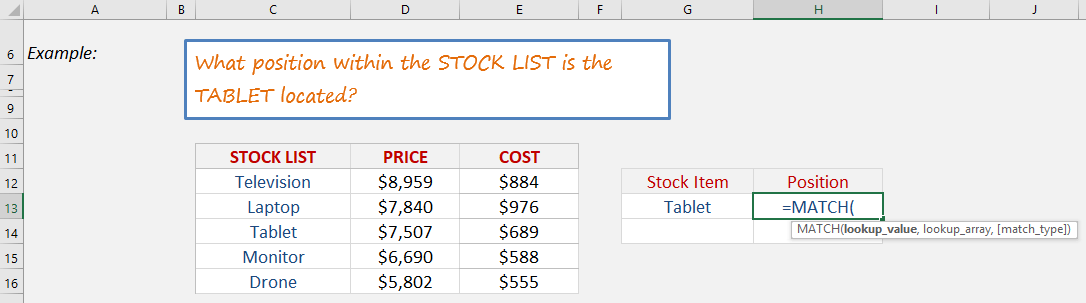STEP 2: The MATCH arguments:

## Lookup_value

What is the value that we want to match?
We want to match the Tablet.

## =MATCH(G13,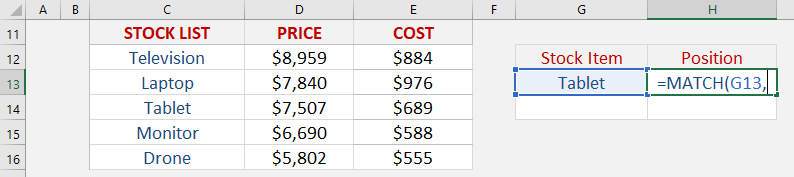## Lookup_array

Where is the list that contains the stock items?

## =MATCH(G13, C12:C16,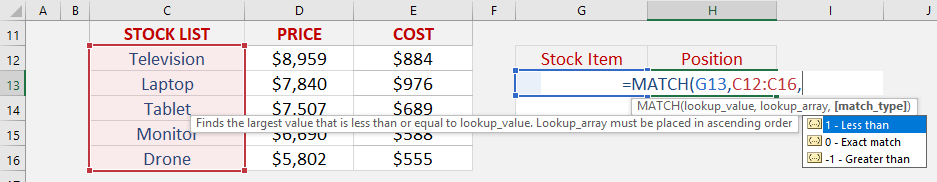## Match_type

What kind of matching do you want?
Let’s put in 0 to get the exact match

## =MATCH(G13, C12:C16, 0)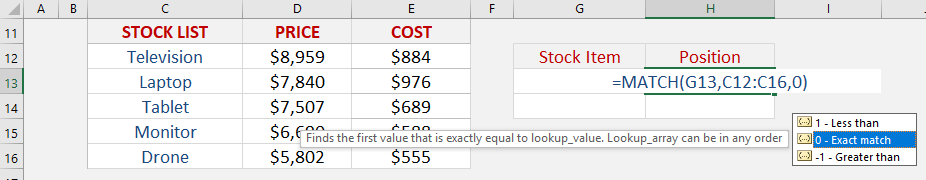And with that, you will get that tablet is in Row #3!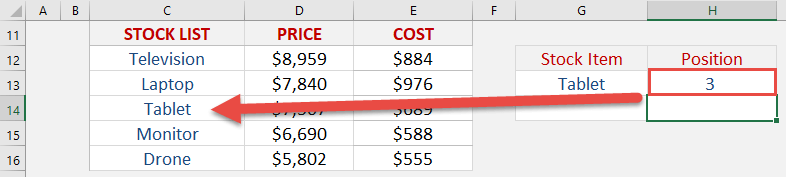## Match Two Lists With The MATCH Function

What does it do?
It returns the position of an item in a range
Formula breakdown:
=MATCH(lookup_valuelookup_array[match_type])
What it means:
=MATCH(lookup this valuefrom this list or range of cellsreturn me the Exact Match).

I am sure that you have come across many occasions where you have two lists of data and want to know if a specific item in List1 exists in List2.
Well I have!
With the MATCH function you can verify if a cell´s item in List1 exists in List2.
The function will return the row position of that item in List2 hence confirming that it exists.  If you get a #N/A it means that the cell´s item does not exist in List2.
You can then go ahead and filter your List1 with either the values returned or the #N/As.
Here are our 2 Lists: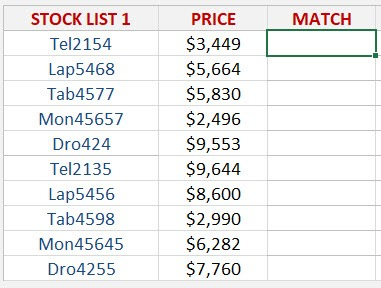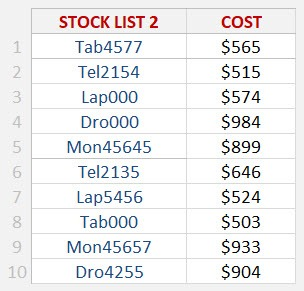STEP 1: We need to enter the MATCH function in a blank cell:

## =MATCH(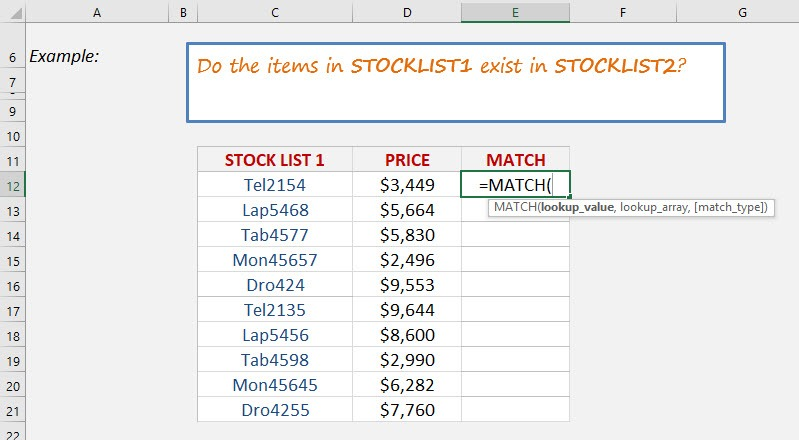STEP 2: The MATCH arguments:

## Lookup_value

What is the value you want to check?
Select the cell containing the List1 value, as this is what we want to check against List2.

## =MATCH(C12,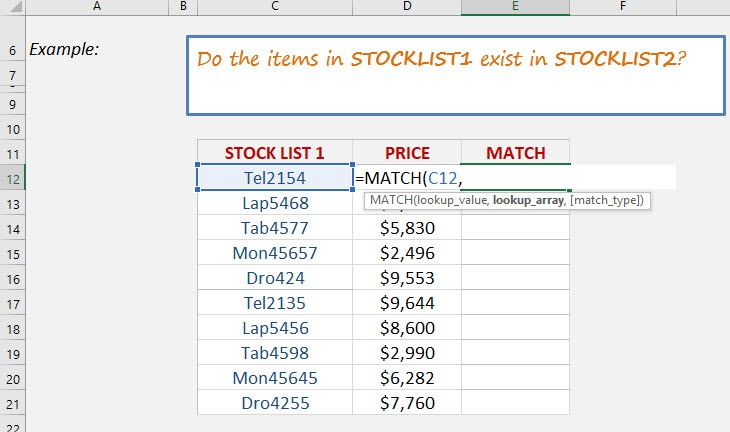## Lookup_array

What is the list you want to check against?
Select the entire List2.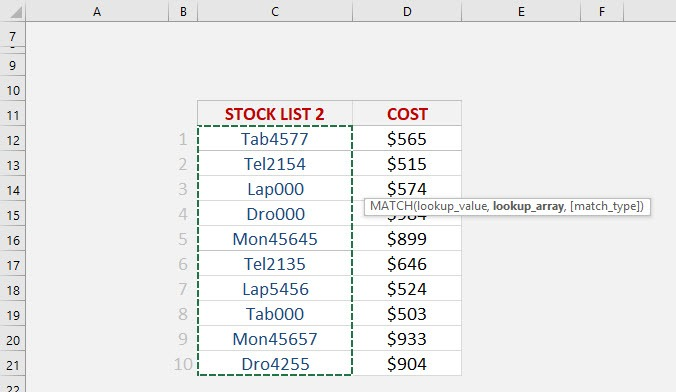And ensure to press F4 to make it an absolute reference.

## =MATCH(C12, list2!\$C\$12:\$C:21,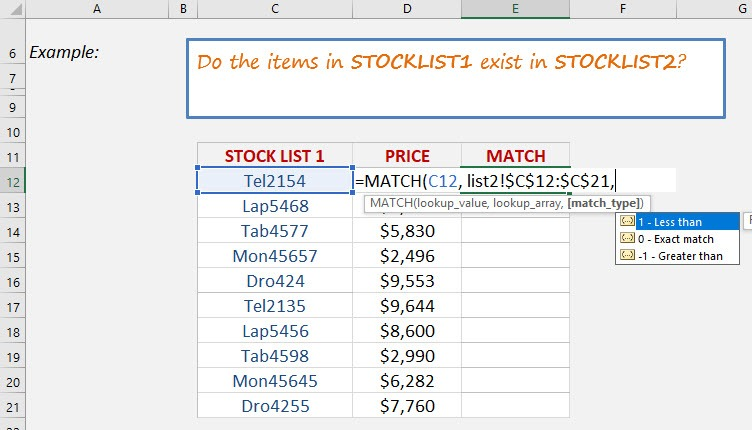## Match_type

We want an exact match so place in 0.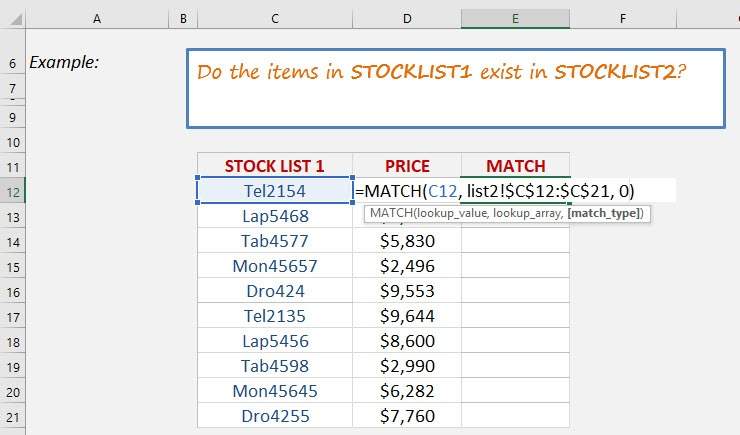Apply the same formula to the rest of the cells by dragging the lower right corner downwards.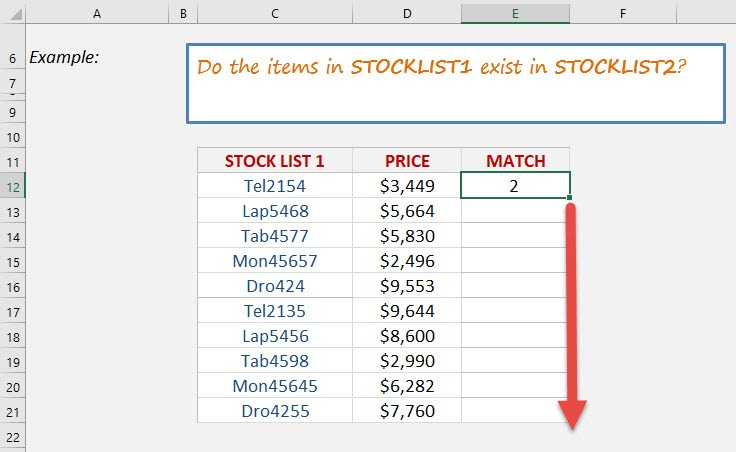You now have all of results! You can see which row numbers the items exist in List2. For example, Mon45657 in List1 exists in List2 Row 9! If it does not exist in List2, then #N/A is displayed.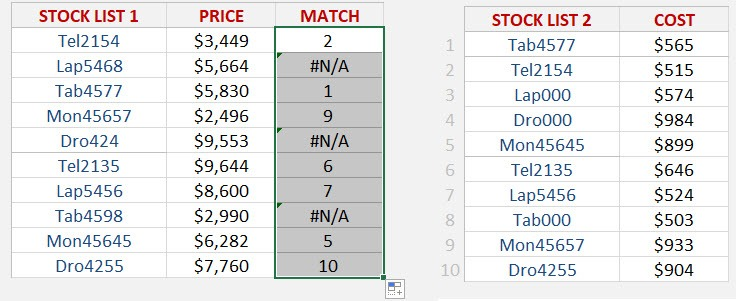## How To Use INDEX-MATCH Formula

What does it do?
Searches the row position of a value/text in one column (using the MATCH function)
and returns the value/text in the same row position from another column to the left or right (using the INDEX function)
Formula breakdown:
=INDEX(array, MATCH(lookup_value, lookup_array, [match_type])
What it means:
=INDEX(return the value/text, MATCH(from the row position of this value/text))

The VLOOKUP formula searches for a value in the first column of an array and returns a value to the right of that array.
How about if you wanted to return a value to the left hand side of that array?
Well, this is where the INDEX-MATCH formula comes in and gives you a helping hand!
It searches the row position of a value/text in one column (using the MATCH function) and returns the value/text in the same row position from another column to the left or right (using the INDEX function).

We want to get the stock id of the tablet, and we will use a combination of INDEX and MATCH to get this!
STEP 1: We need to enter the INDEX function in a blank cell:

## =INDEX(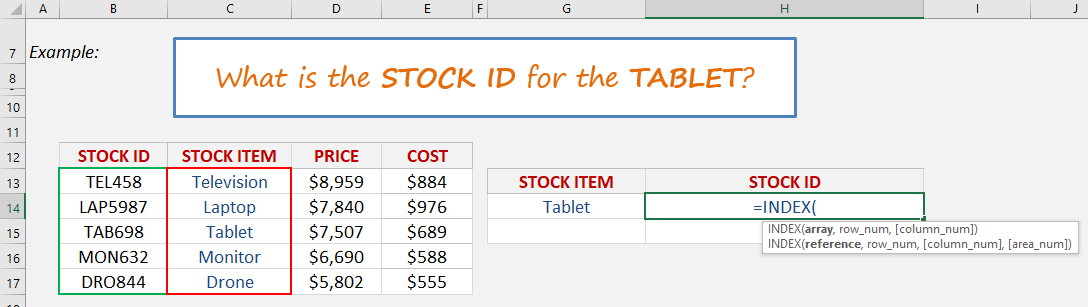STEP 2: The INDEX arguments:

## Array

Where is the list that contains the stock ids?

## =INDEX(B13:B17,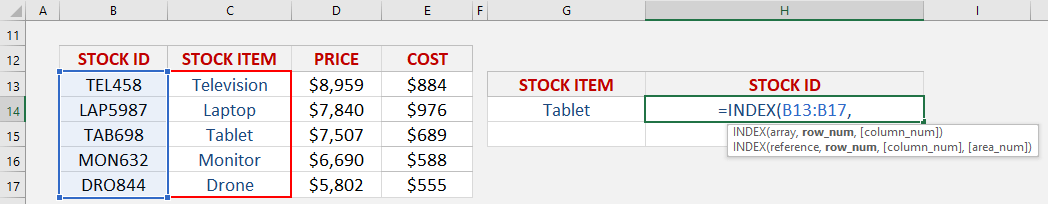## Row_num

What row number contains the data?
Let us use the Match function to get the row number.

## =INDEX(B13:B17, MATCH(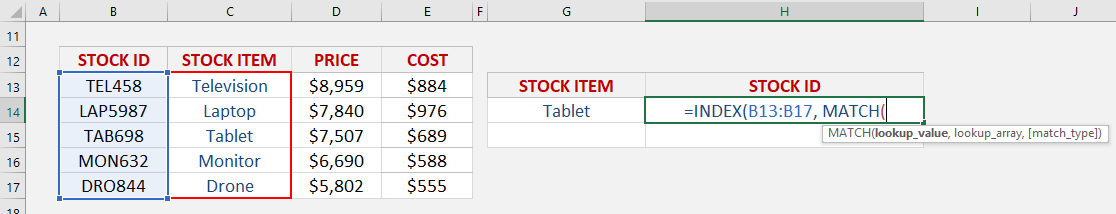STEP 3: The MATCH arguments:

## Lookup_value

What is the value that we want to match?
We want to match the Tablet.

## =INDEX(B13:B17, MATCH(G14,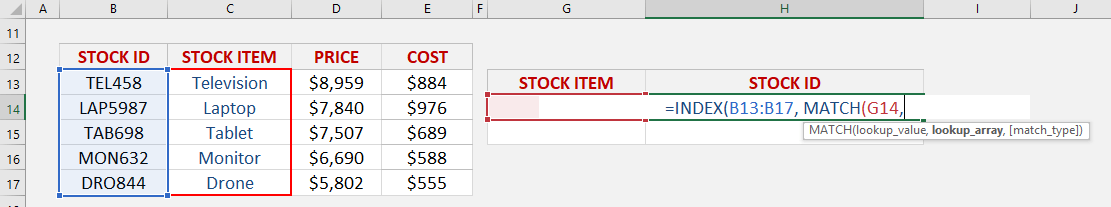## Lookup_array

Where is the list that contains the stock items?

## =INDEX(B13:B17, MATCH(G14, C13:C17,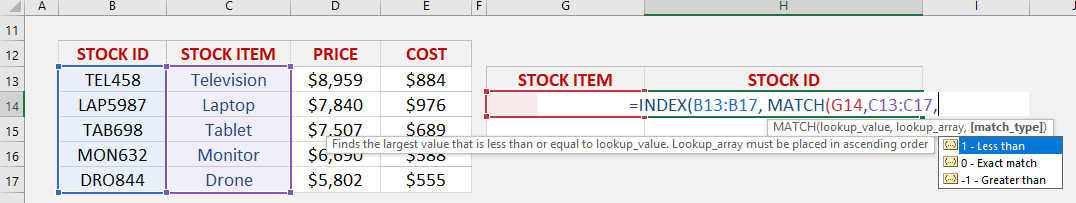## Match_type

What kind of matching do you want?
Let’s put in 0 to get the exact match

## =INDEX(B13:B17, MATCH(G14, C13:C17, 0))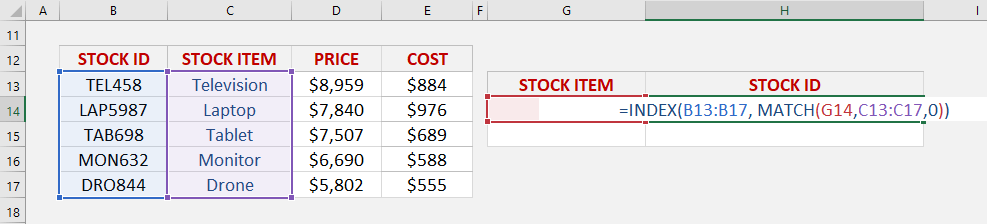With this, the MATCH function will get the row number containing the Tablet, which is row #3. Then with Row #3, we will get the stock id in that same row using the INDEX function.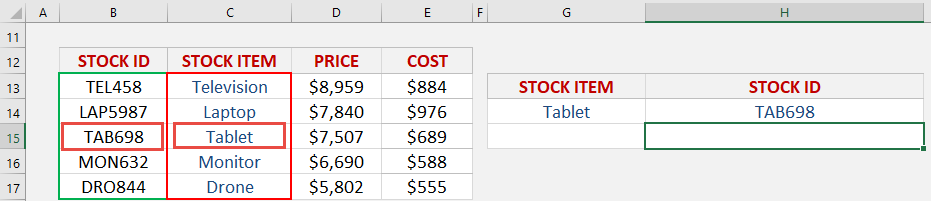Latest Tutorials

February 1, 2021

August 18, 2015